# 看完这篇 HashMap ，和面试官扯皮就没问题了（3）

+关注继续查看

### HashMap 构造函数

• 带有初始容量 initialCapacity负载因子 loadFactor 的构造函数

public HashMap(int initialCapacity, float loadFactor) {
if (initialCapacity < 0)
throw new IllegalArgumentException("Illegal initial capacity: " +
initialCapacity);
if (initialCapacity > MAXIMUM_CAPACITY)
initialCapacity = MAXIMUM_CAPACITY;
throw new IllegalArgumentException("Illegal load factor: " +
// 扩容的阈值
this.threshold = tableSizeFor(initialCapacity);
}

• 只带有 initialCapacity 的构造函数

public HashMap(int initialCapacity) {

}

• 无参数的构造函数

public HashMap() {  this.loadFactor = DEFAULT_LOAD_FACTOR;}

• 带有 map 的构造函数

public HashMap(Map<? extends K, ? extends V> m) {  this.loadFactor = DEFAULT_LOAD_FACTOR;  putMapEntries(m, false);}

### 讲一讲 HashMap put 的全过程

final V putVal(int hash, K key, V value, boolean onlyIfAbsent,                   boolean evict) {  Node<K,V>[] tab; Node<K,V> p; int n, i;  // 如果table 为null 或者没有为 table 分配内存，就resize一次  if ((tab = table) == null || (n = tab.length) == 0)    n = (tab = resize()).length;  // 指定hash值节点为空则直接插入，这个(n - 1) & hash才是表中真正的哈希  if ((p = tab[i = (n - 1) & hash]) == null)    tab[i] = newNode(hash, key, value, null);  // 如果不为空  else {    Node<K,V> e; K k;    // 计算表中的这个真正的哈希值与要插入的key.hash相比    if (p.hash == hash &&        ((k = p.key) == key || (key != null && key.equals(k))))      e = p;    // 若不同的话，并且当前节点已经在 TreeNode 上了    else if (p instanceof TreeNode)      // 采用红黑树存储方式      e = ((TreeNode<K,V>)p).putTreeVal(this, tab, hash, key, value);    // key.hash 不同并且也不再 TreeNode 上，在链表上找到 p.next==null    else {      for (int binCount = 0; ; ++binCount) {        if ((e = p.next) == null) {          // 在表尾插入          p.next = newNode(hash, key, value, null);          // 新增节点后如果节点个数到达阈值，则进入 treeifyBin() 进行再次判断          if (binCount >= TREEIFY_THRESHOLD - 1) // -1 for 1st            treeifyBin(tab, hash);          break;        }        // 如果找到了同 hash、key 的节点，那么直接退出循环        if (e.hash == hash &&            ((k = e.key) == key || (key != null && key.equals(k))))          break;        // 更新 p 指向下一节点        p = e;      }    }    // map中含有旧值，返回旧值    if (e != null) { // existing mapping for key      V oldValue = e.value;      if (!onlyIfAbsent || oldValue == null)        e.value = value;      afterNodeAccess(e);      return oldValue;    }  }  // map调整次数 + 1  ++modCount;  // 键值对的数量达到阈值，需要扩容  if (++size > threshold)    resize();  afterNodeInsertion(evict);  return null;}

• hash -> put 放在桶中的位置，在 put 之前，会进行 hash 函数的计算。
• key -> 参数的 key 值
• value -> 参数的 value 值
• onlyIfAbsent -> 是否改变已经存在的值，也就是是否进行 value 值的替换标志
• evict -> 是否是刚创建 HashMap 的标志

public V put(K key, V value) {  return putVal(hash(key), key, value, false, true);}

static final int hash(Object key) {  int h;  return (key == null) ? 0 : (h = key.hashCode()) ^ (h >>> 16);}

#### Hash 函数

hash 函数会根据你传递的 key 值进行计算，首先计算 key 的 hashCode 值，然后再对 hashcode 进行无符号右移操作，最后再和 hashCode 进行异或 ^ 操作。

>>>: 无符号右移操作，它指的是 「无符号右移，也叫逻辑右移，即若该数为正，则高位补0，而若该数为负数，则右移后高位同样补0」 ，也就是不管是正数还是负数，右移都会在空缺位补 0 。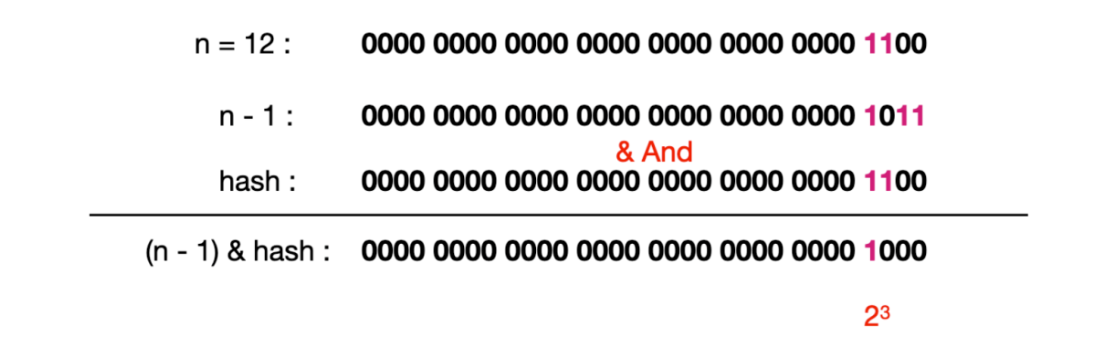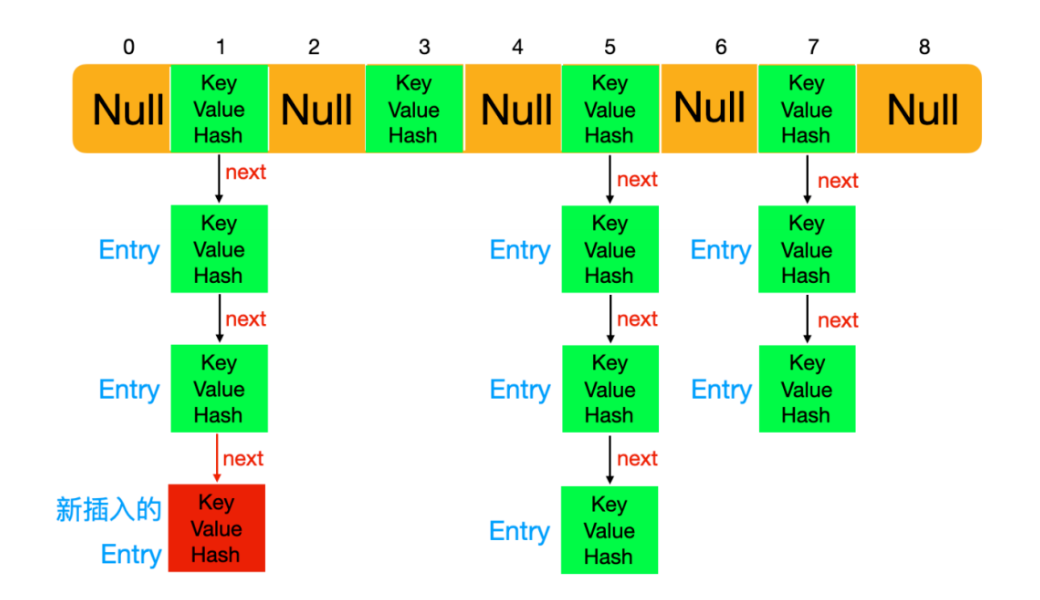### 扩容机制

final Node<K,V>[] resize() {  Node<K,V>[] oldTab = table;  // 存储old table 的大小  int oldCap = (oldTab == null) ? 0 : oldTab.length;  // 存储扩容阈值  int oldThr = threshold;  int newCap, newThr = 0;  if (oldCap > 0) {    // 如果old table数据已达最大，那么threshold也被设置成最大    if (oldCap >= MAXIMUM_CAPACITY) {      threshold = Integer.MAX_VALUE;      return oldTab;    }    // 左移扩大二倍,    else if ((newCap = oldCap << 1) < MAXIMUM_CAPACITY &&             oldCap >= DEFAULT_INITIAL_CAPACITY)      // 扩容成原来二倍      newThr = oldThr << 1; // double threshold  }  // 如果oldThr                                                                                                                                               !> 0  else if (oldThr > 0) // initial capacity was placed in threshold    newCap = oldThr;  // 如果old table <= 0 并且 存储的阈值 <= 0  else {               // zero initial threshold signifies using defaults    newCap = DEFAULT_INITIAL_CAPACITY;    newThr = (int)(DEFAULT_LOAD_FACTOR * DEFAULT_INITIAL_CAPACITY);  }  // 如果扩充阈值为0  if (newThr == 0) {    // 扩容阈值为 初始容量*负载因子    float ft = (float)newCap * loadFactor;    newThr = (newCap < MAXIMUM_CAPACITY && ft < (float)MAXIMUM_CAPACITY ?              (int)ft : Integer.MAX_VALUE);  }  // 重新给负载因子赋值  threshold = newThr;  // 获取扩容后的数组  @SuppressWarnings({"rawtypes","unchecked"})  Node<K,V>[] newTab = (Node<K,V>[])new Node[newCap];  table = newTab;  // 如果第一次进行table 初始化不会走下面的代码  // 扩容之后需要重新把节点放在新扩容的数组中  if (oldTab != null) {    for (int j = 0; j < oldCap; ++j) {      Node<K,V> e;      if ((e = oldTab[j]) != null) {        oldTab[j] = null;        if (e.next == null)          newTab[e.hash & (newCap - 1)] = e;        else if (e instanceof TreeNode)          // 重新映射时，需要对红黑树进行拆分          ((TreeNode<K,V>)e).split(this, newTab, j, oldCap);        else { // preserve order          Node<K,V> loHead = null, loTail = null;          Node<K,V> hiHead = null, hiTail = null;          Node<K,V> next;          // 遍历链表，并将链表节点按原顺序进行分组          do {            next = e.next;            if ((e.hash & oldCap) == 0) {              if (loTail == null)                loHead = e;              else                loTail.next = e;              loTail = e;            }            else {              if (hiTail == null)                hiHead = e;              else                hiTail.next = e;              hiTail = e;            }          } while ((e = next) != null);          // 将分组后的链表映射到新桶中          if (loTail != null) {            loTail.next = null;            newTab[j] = loHead;          }          if (hiTail != null) {            hiTail.next = null;            newTab[j + oldCap] = hiHead;          }        }      }    }  }  return newTab;}

• 判断 HashMap 中的数组的长度，也就是 (Node<K,V>[])oldTab.length() ，再判断数组的长度是否比最大的的长度也就是 2^30 次幂要大，大的话直接取最大长度，否则利用位运算 <<扩容为原来的两倍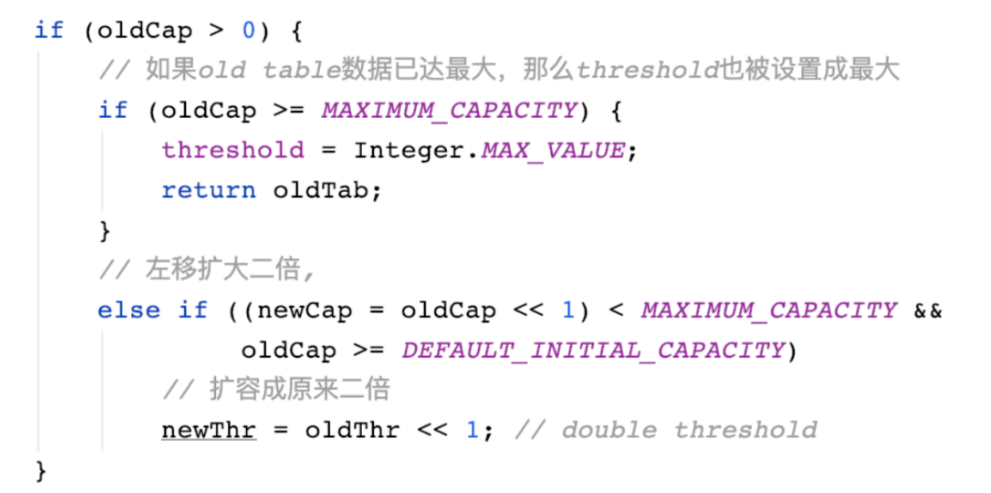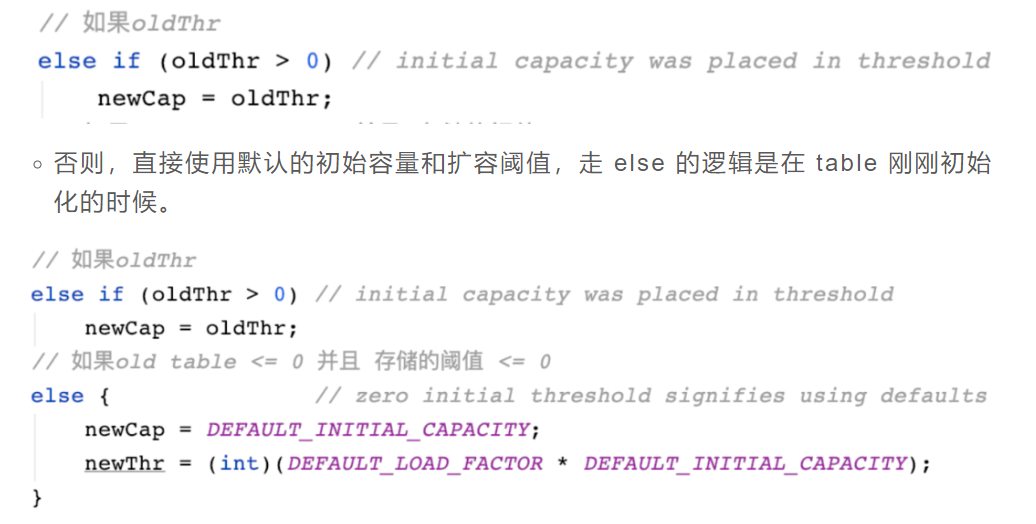「在扩容后需要把节点放在新扩容的数组中，这里也涉及到三个步骤」

• 循环桶中的每个 Node 节点，判断 Node[i] 是否为空，为空直接返回，不为空则遍历桶数组，并将键值对映射到新的桶数组中。

• 如果不为空，再判断是否是树形结构，如果是树形结构则按照树形结构进行拆分，拆分方法在 split 方法中。

• 如果不是树形结构，则遍历链表，并将链表节点按原顺序进行分组。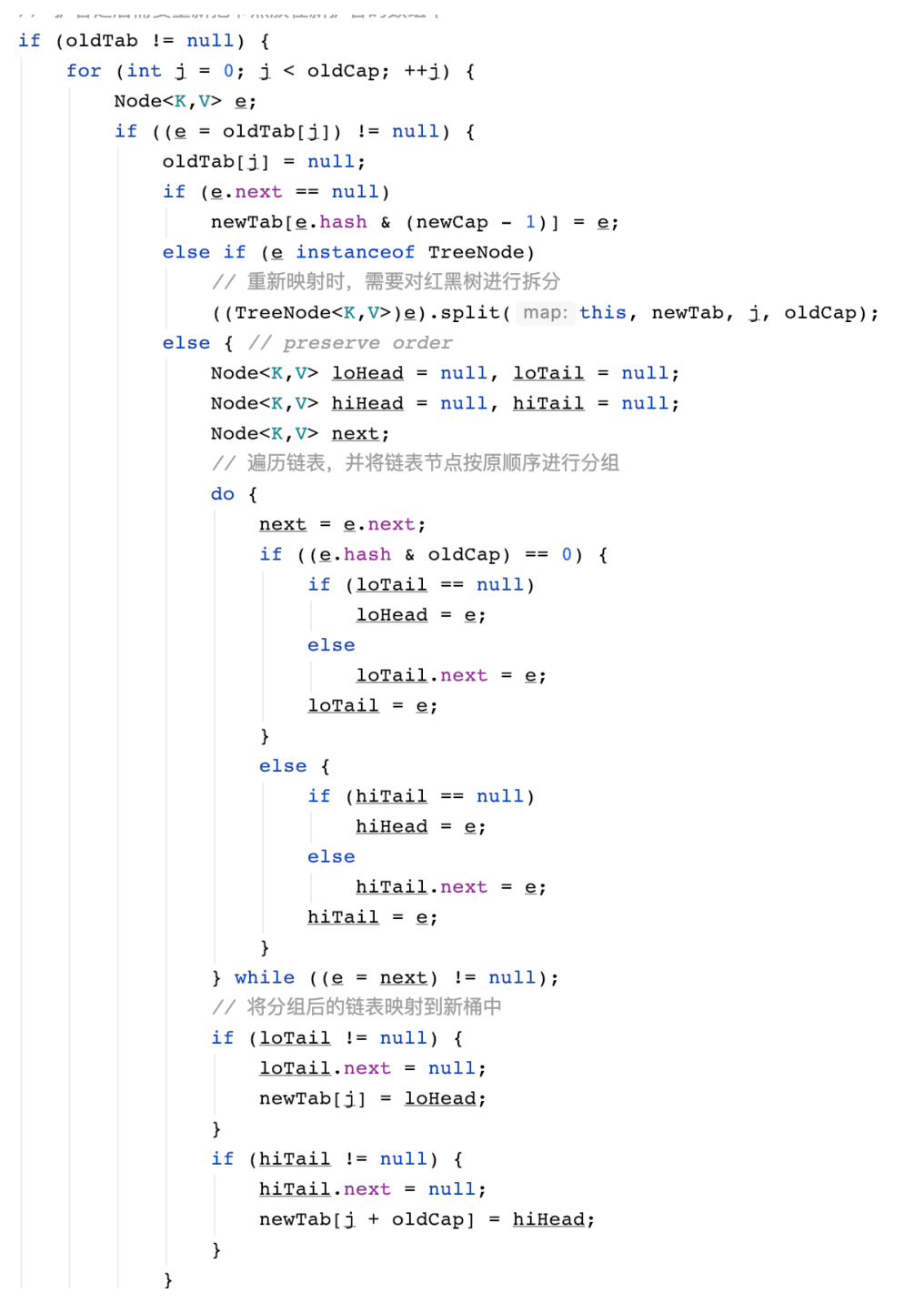</div>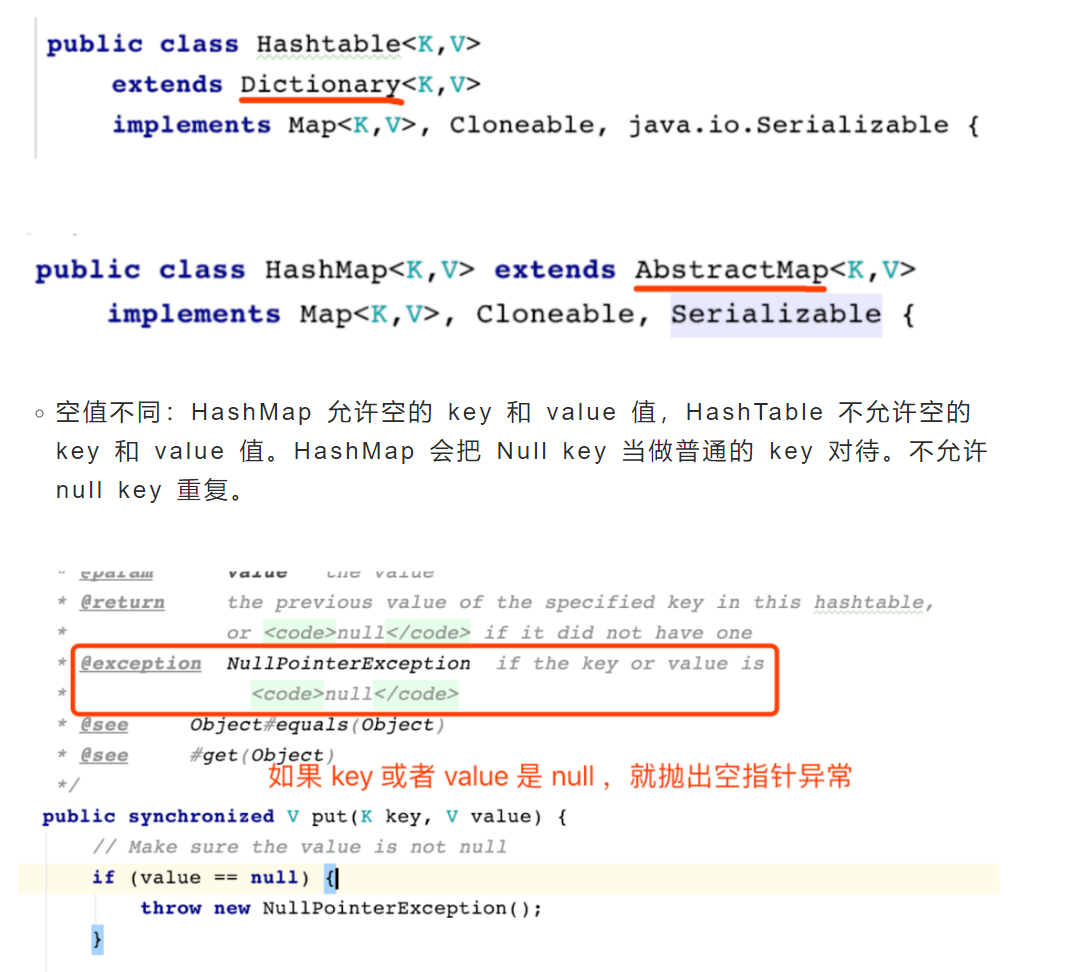18 0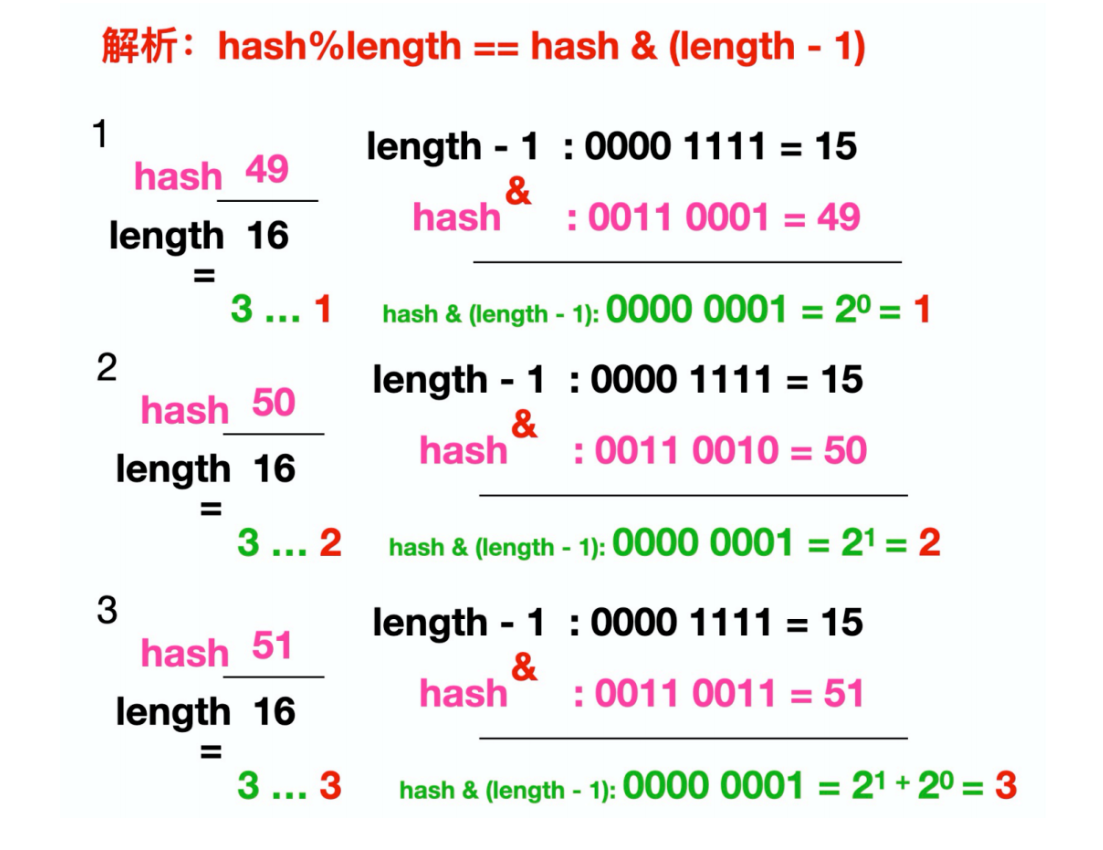10 014 07 0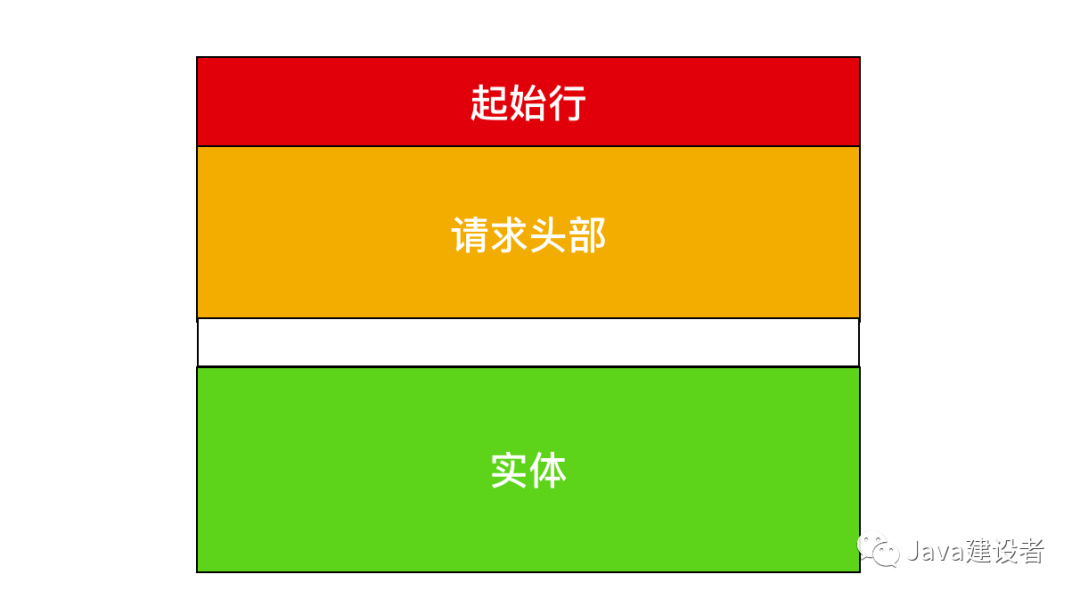14 09 023 015 0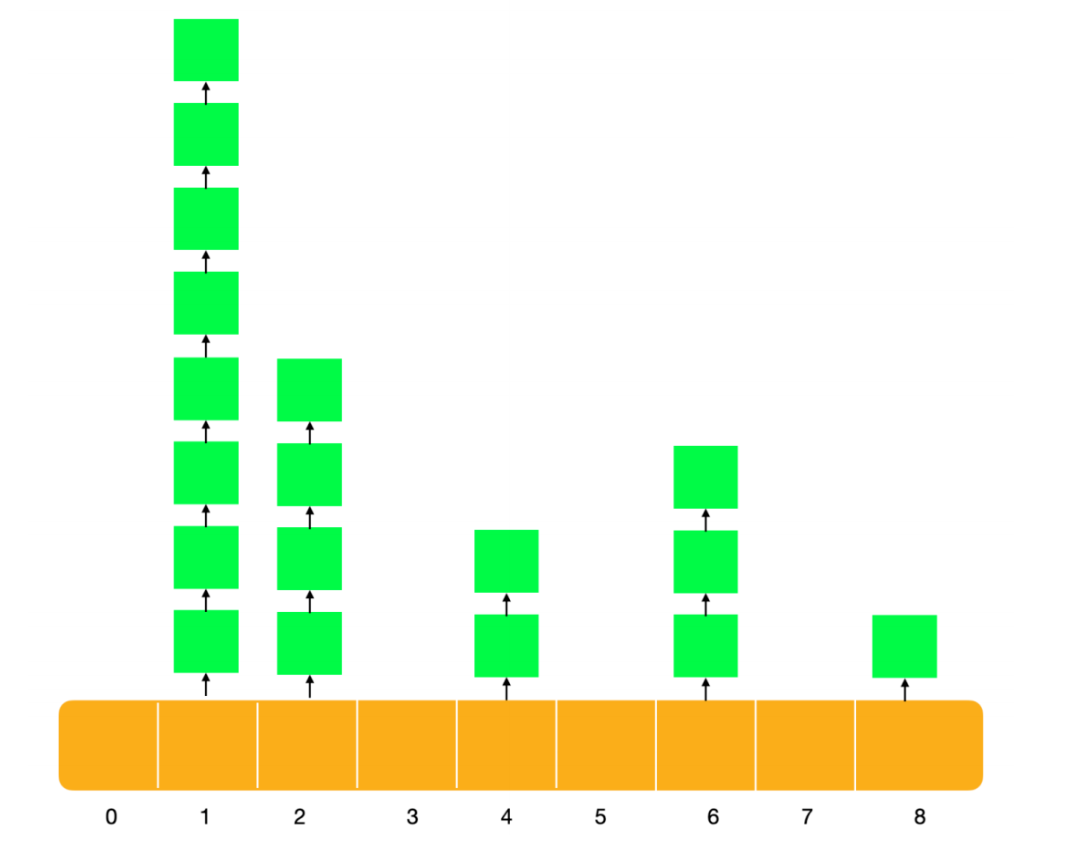37 058 0+关注
3238

0